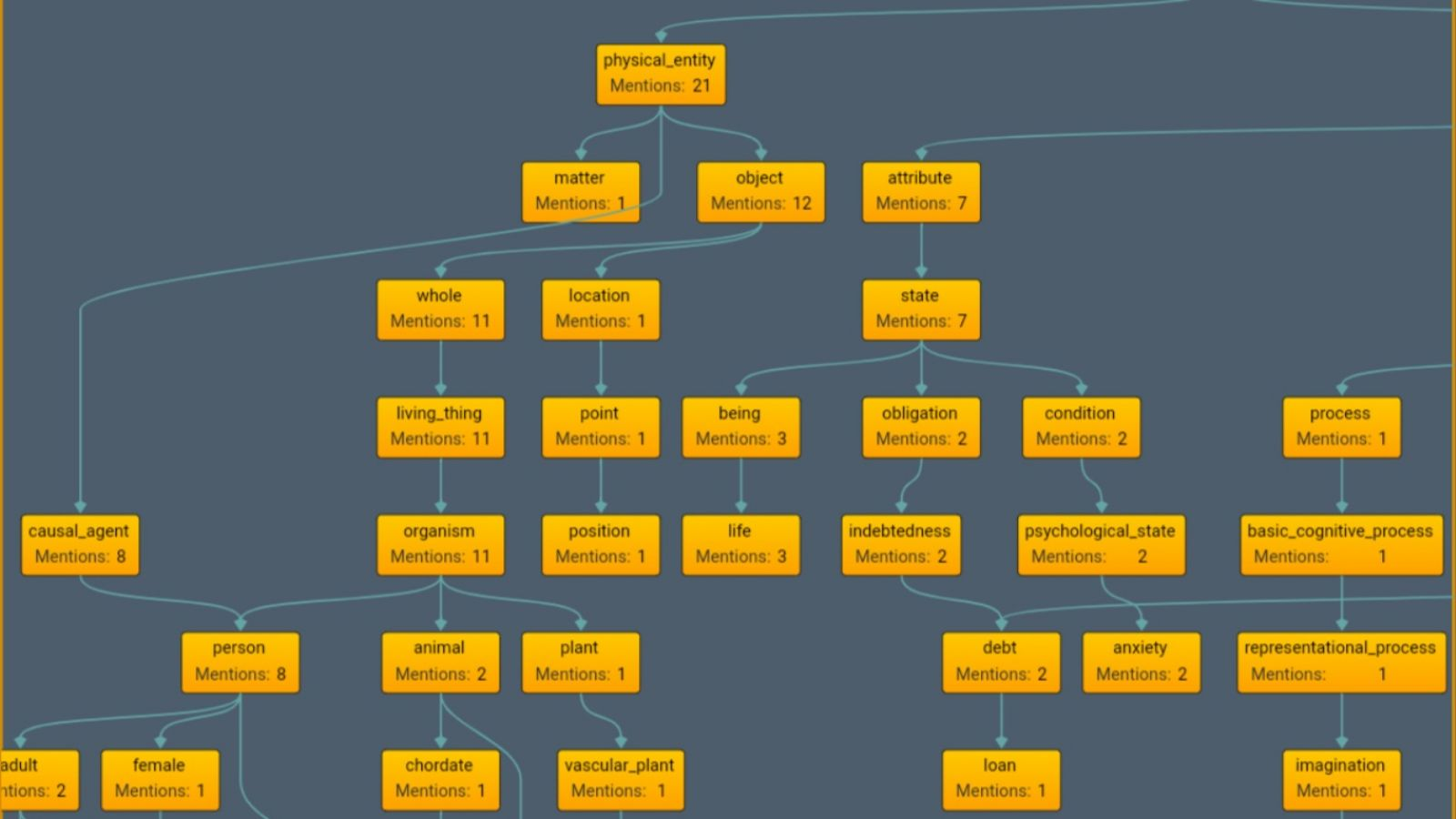# Tauchain: More thoughts on software as formulas, as well as thoughts on human computation and program synthesis

One of the important concepts I discussed in the previous post will form the basis for my discussion today. In the previous post:

program: f(x) { return x + x }
formula: S(x,y): = y=x+x

To explain this in a bit more detail, we know generally what a function is from mathematics but for those who do not know, a function takes some input, crunches numbers according to a formula, and then produces an output. A program essentially is the same where the user gives the program some input (data) which applies to some formulas which then transforms or produces the output (data).

We know the quality of the output is based on the quality of the input. To bring this into context I will give a few examples:

RiskFactors = (ln(Age) * AgeFactor) + (ln(TotalChol) * TotalCholFactor) + (ln(HDLChol) * HDLCholFactor) + (ln(SysBP) * SysBPFactor) + Cig + DM - AvgRisk
Risk = 100 * (1 - RiskPeriodFactore(RiskFactors))

The formula you see here is the the formula for the Framingham Risk Score. This score is based on the data the user or the doctor inputs in. The formula crunches the numbers and outputs a score which doctors then use to guide their treatment decisions along a logical decision algorithm.

Now a more simple formula which which any medical student would know is:

Creatinine Clearance = [[140 - age(yr)]weight(kg)]/[72serum Cr(mg/dL)] (multiply by 0.85 for women)**

Now, the formula is known. In Tau we can just systematically add known formulas (knowledge), or if we are creative we can create new formulas. Suppose we add this? In order to work with a formula like this Tau would need to be able to handle arithmetic but lets assume it can? Well the input is sent as data to this formula or program and the output is the result.

The problem? The ability to get good results to guide the decision of the doctor requires that the data being input is confirmed to be true. In medicine this is lab blood test results taken over time (medical history) etc. In the situation we rely on data with Tau one potential problem is how can we trust the results unless we can trust the input data? If the users input bogus data the software will work just fine according to formal verification etc, but the data itself could be untrue which would make the result untrue.

If it is important decisions to be made involving risk then we need a way for the community to determine the data is true with a high degree of certainty. How would we achieve that? That is an open question for the community. Program synthesis is really neat in that it can improve productivity by reducing the effort in coding and allowing more focus to be on actually applying knowledge we have (formulas we know) or in actually augmenting our decisions by applying the results of the calculations from this application of knowledge.

If you have access to high quality data or if you are the source of your own input data then you can know using Tau that your output is correct. The Tau cannot be hacked in the traditional sense of bug in the code but there can be a bug in the user or in the data.

Human computation, human computers, this is the basis behind Proof of Brain on Steem. Humans essentially agree to sell their brain power for the benefit of the network. There are many tasks which human brain power can compute fast but a robot cannot. The human computer is not a new concept and traditionally the humans who broke codes or who were hackers, were called "human computers". Many of these humans were women who did it in a security or military context during war. Today the human computer concept now is "human computation" and it includes Amazon Mechanical Turk, Steem manual curation also called collaborative filtering, etc.

Human computation involves the human applying custom algorithms and heuristics toward producing a score or ranking or other quantifiable output. Collaborative filtering is in essence not that different from the software example above but the difference is each human can come up with their own formula or just let their subconscious determine it. Subjective value can be quantified and calculated consciously or it can be subconsciously determined. In my opinion Tau and Agoras could benefit from leveraging human computation and this could also expand beyond Proof of Brain which we see in Steem.

3

Previous Next

### Recent post